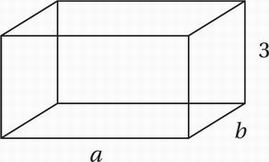# SAT Math Multiple Choice Question 605: Answer and Explanation

### Test Information

Question: 605

5.Note: Figure not drawn to scale

A rectangular solid above has dimensions 3, a, and b, where a and b are integers. Which of the following CANNOT be the areas of three different faces of this solid?

• A. 15, 18, and 30
• B. 18, 24, and 48
• C. 12, 15, and 24
• D. 15, 24, and 40

Explanation:

C

Special Topics (three-dimensional geometry) MEDIUM

On the drawing, we should first mark the areas of the three faces. The front and back faces both have an area of 3a. The left and right faces both have an area of 3b. The top and bottom faces both have an area of ab. We should now try to find integer values for a and b so that these areas match those given in the choices.

(A) 15, 18, and 30

This is possible if a = 5 and b = 6.

(B) 18, 24, and 48

This is possible if a = 6 and b = 8.

(C) 12, 15, and 24

This cannot work for any integer values of a and b.

(D) 15, 24, and 40

This is possible if a = 5 and b = 8.